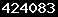# Posts Tagged ActiveWorkbook

### Write Data From DataTable To Excel With an Array Using C#

This is actually a redo of code written in vb.net a while back that dumps data from a datatable in a dataset into an excel spreadsheet in an effective and fast manner. I am always getting asked how to do this in C# so I decided to put that up today. Yes, I do C# too! Just didn’t want to admit it….

It’s pretty self explanatory. Don’t forget to clean up your excel instance when done. If you have any questions please feel free to send me an email.

Import an excel reference and at the top of your code you need your import statements. Then simply pass your dataset and datatable (granted you could just pass the datatable – I had my reasons for passing the dataset too at the time) to the method. The array is declared as an object because sometimes the compiler has to deal with unforeseen data issues that might arise and it has been effective for me to do let it handle those situations as they arise. I hope you find this useful.

using Excel = Microsoft.Office.Interop.Excel;
using Office = Microsoft.Office.Core;

public void CreateSpreadSheetFromDataSet(DataSet ds, DataTable dt)
{

Excel.Application Excel = new Excel.Application();
Excel.Visible = true;
Excel.Worksheet WSheet = new Excel.Worksheet();
WSheet = Excel.ActiveWorkbook.ActiveSheet;
int rows = ds.Tables[dt.TableName].Rows.Count;
int columns = ds.Tables[dt.TableName].Columns.Count;
int r = 0; int c = 0;
object[,] DataArray = new object[rows + 1, columns + 1];
for (c = 0; c <= columns – 1; c++)
{
DataArray[r, c] = ds.Tables[dt.TableName].Columns[c].ColumnName;
for (r = 0; r <= rows – 1; r++)
{
DataArray[r, c] = ds.Tables[dt.TableName].Rows[r][c];
} //end row loop
} //end column loop

//actually write array to Excel Spreadsheet
WSheet.Range[“A2”].Resize[rows, columns].Value = DataArray;